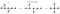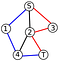# The Proof of Wagner’s Conjecture and Why it Matters

I have always found it fascinating how fundamental results from math can be applied to solve concrete computational problems that I encounter when writing software. However, while I love math I often find that many recent results are increasingly abstract and not in any way applicable to problems that I can imagine running into. This is how I felt when I heard about a series of 20+ papers, published by Neil Robertson and Paul Seymour, which in 2004 managed to prove Wagner’s Conjecture, a combinatorial result that “any infinite list of finite undirected graphs contains at least one graph that is a minor of another graph in the list”.

While it is true that finite undirected graphs are seen everywhere in software, from social networks to flow graphs and everything in between, it is rare to encounter a problem dealing with an infinite list of these graphs, making this result initially seems occult and non-applicable to the real world. However, as I discovered and aim to show you, the consequences of this result have opened the door to a whole new class of solutions to some very common (and previously very hard) graph problems.

# What is a Graph Minor?

Before we dive into the applications of the theorem we must first understand what it is saying, and to do that we need to know what a graph-minor is. Luckily there is nothing complicated about this. A graph minor H of some other graph G, is simply the graph which is the result of contracting (merging 2 vertexes that share an edge into one vertex) or deleting any number of vertexes and edges in G.The process of making a minor H from G. From http://en.wikipedia.org/wiki/Graph_minor

Looking at this property it is clear that any finite graph has a finite number of minors (there is a limit to how much stuff we can contract/remove from a graph to make new minors). Furthermore, every graph has a unique set of minors associated with it. With this understanding of minors in mind if we look back at Wagner’s Conjecture, it is saying that given an infinite number of finite undirected graphs, there will be at least one graph that you can contract and remove vertexes from to make it look like another graph on that infinite list.

This conjecture is still abstract and knowing what a minor is doesn't really help explain how the result can be useful. To understand that we will have to go back to the inspiration for Wagner’s Conjecture.

# Where did Wagner’s Conjecture Come From?

The conjecture was proposed by the German graph theorist Klaus Wagner in the 1930's (formally in a 1970 paper) after he made a discovery about planar graphs.

What is a planar graph? Well simply put a graph (represented by a set of connections between vertexes) is planer if it can be drawn on a piece of paper (a 2D plane) with no edges intersecting. For example if you want to draw a graph consisting of 5 vertexes in which each vertex is connected to every other vertex there is no possible way to do this without 2 edges intersecting, even if the edges aren't drawn straight, meaning that this graph is non-planar.

What Wagner noticed was that ANY graph that is planer does not have the graphs below as minors

(for you math people these graphs are the complete graph on 5 vertexes or a K5, and the complete bipartite graph on 6 vertexes or a K3,3). Furthermore any graph that does not contain these so-called “forbidden minors” is necessarily planer.

Now this result seems much more applicable to a real world problem programmers could encounter such as: “Given a graph tell me if I can render it on a screen without the edges crossing”. However, Wagner took this result even further when he posited that when drawing graphs any surface, such as a the surface of a sphere, or a 2D plane, or a 3D doughnut (seen below),

there will be a unique finite set of “forbidden minors” that define what graphs can be drawn on these surfaces without their edges crossing (known as being embedded on the surface). This generalization, if true, would be a huge result in and of itself but Wagner took it one step further.

This last leap to get to the conjecture is where it gets a bit complicated, so bear with me. Lets assume any fixed surface has a unique finite set of forbidden minors, lets call this S. We know that there is no graph G1 in S that is a minor of any other graph G2 in S, since if there were then we could remove G2 from S without changing if S correctly classifies graphs as embeddable in the surface (think about this for a second). Because of this property, S will only be finite for every surface is if it were not possible to build an infinite set of graphs where no two are minors of one another. This is where the conjecture comes from, since the conjecture being true would imply the existence of a finite set of forbidden minors defining what graphs are embeddable on what surfaces.

A more math-y way to think about the conjecture is by defining a poset under the minor relation on the space of all finite graphs. Then S must be an anti-chain in this poset and the conjecture simply states that there do not exist infinite length anti-chains in this poset.

OK so now we know the practical underpinnings that led Wagner to make his conjecture, but beyond testing for embeddable graphs what does the truth of this conjecture allow us to do?

# What does Wagner’s Conjecture Being True Mean?

As a quick note, the proof of Wagner’s conjecture/Graph Structure Theorem is extremely complex (over 600 pages long) and will not be covered in this paper. We will take major results as a given.

Without picking apart the recent proof of Wagner’s Conjecture too much, one of the key results that falls out is an cubic time way to test if any graph G1 is a minor of any other graph G2 (cubic in the number of vertexes in G2). Taking this along with the truth of the conjecture we can see that because there are a finite and fixed number of minors describing what can and can’t be embedded on a given surface, we can use this cubic algorithm to test if these forbidden-minors are minors of a given graph G, yielding a polynomial time algorithm for testing embeddability of any graph G on any arbitrary surface. Again this is a cool result and potentially useful to programmers in solving some more abstract problems but it is only the tip of the iceberg.

Consider for a moment a common problem in graph theory and computer science: The Disjoint Path Problem. The problem gives you a graph G and a set of K pairs of vertexes {(S1, T1), (S2, T2)… (Sk, Tk)}, the goal is to find K paths on the graph between each S and T pair such that none of the paths share vertexes (other than possibly their start and end vertexes) or conclude that such paths do not exist.A simple solution to the disjoint path problem where S1=S2 and T1=T2 from http://qph.is.quoracdn.net/main-qimg-92470d189339544e6b673b8e5c0f49bd?convert_to_webp=true

This problem appears in many domains, from network analysis to VLSI design. As an example checking for redundancy in the connections between servers on a network like the internet is an instance of the disjoint path problem.

Before the proof of Wagner’s conjecture there existed algorithms for solving this problem for K=1 (a simple graph search) and K=2, but the problem only had a non-polynomial solution for any larger fixed K. However, cleverly applying a result of Wagner’s conjecture lets us improve significantly on this.

Consider we have been given the disjoint path problem and have already found the K disjoint paths in our graph G. We can now make a minor of G by contracting the edges along every path we found to make each S connected directly to its corresponding T. This will yield a minor graph containing K distinct edges connecting the T’s and S’s. There are finitely many minors of this form for our graph G, lets call this set of minors M. With our cubic algorithm for checking if one graph is a minor of another it is intuitively possible to check if elements in M are minors of G, and hence show which paths in G can be compressed to connect the K pairs of vertexes via disjoint paths (or if none of the elements in M are minors of G conclude it is impossible to find K disjoint paths). This is in no way a formal proof or description of the algorithm, but rather an intuition behind the complex cubic algorithm proposed by Robertson and Seymour solve the disjoint path problem for any fixed K.

The actual algorithm and proof relies heavily on ideas involving the tree decomposition of graphs, which is beyond the scope of this article but you can read about it here.

This disjoint path solution is a huge result derived from the proof of Wagner’s conjecture, and it has provided programmers with an effective polynomial way to solve problems that were previously exponentially hard. Of all the results falling out of Wagner’s Conjecture this is perhaps the most important thus far, and understanding where it comes from as well as how to apply it is something that may be extremely useful to developers who work extensively with graphs.

# Why is This Still Exciting?

Beyond producing the two algorithms above for testing embeddability and solving the disjoint path problem, the proof of Wagner’s conjecture has opened up a whole new field of combinatorics known as graph structure theory and it has become clear that there is still much more to be learned from this result. There have recently been papers refining the algorithms discovered in the original conjecture proof, such as a 2012 paper by Kawarabayashi et. Al. that gives a quadratic solution to the disjoint path problem, using the concepts derived from the proof of Wagner’s Conjecture. Furthermore, there are new mathematical results coming out of the the groundwork laid by this theorem every year, some of which may potentially yield new scalable algorithms to attack graph problems that previously were intractable.

I write about technology and society. Harvard College ‘16. Find me at arankhanna.com

## More from Aran Khanna

I write about technology and society. Harvard College ‘16. Find me at arankhanna.com